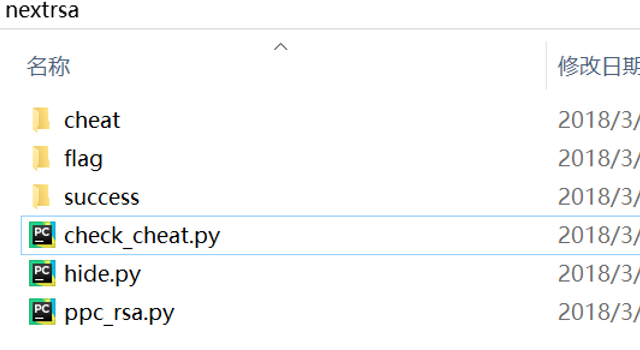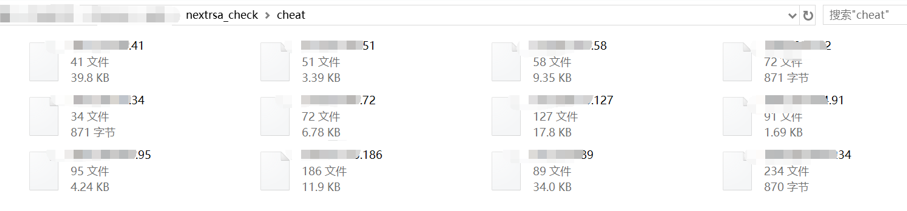## 新闻资讯# 前言

`交互类题目`

#### Nextrsa

Nextrsa这个题目原本思路源自某次比赛的Twin Primes，只有nextprime一个关卡，解题思路也很简单，首先进行假设：

`nextprime(p)*nexprime(q)=(p+x)*(q+y)`

```import gmpy2
ga=gmpy2.mpz(a)
gb=gmpy2.mpz(b)
gc=gmpy2.mpz(c)
delta=gb**2-4*ga*gc
if delta=0 or a==0:
return 0
tmp=gmpy2.iroot(delta,2)
if tmp==True:
x1 = (-gb + tmp) / (2*ga)
x2 = (-gb - tmp) / (2 * ga)
if x1>0 and n%x1==0:
print x1
return x1
if x2>0 and n%x2==0:
print x2
return x2
return 0
def main():
for x in range(1500):
print x
for y in range(1500):
a=y
b=x*y-m+n
c=x*n
return 0
main()```

```problem_brute_256bit(conn)
problem_wiener_attack(conn)
problem_LLL_attack(conn)
problem_np_nq(conn)
problem_e_3_brute(conn)
problem_gcd_attack(conn)
problem_same_n(conn)

9个关卡。本来我构想是大概到15个左右，但是实在是有点忙，9个也够喂饱萌新了，于是题目最终出成了这样。所有给予的信息，都是完整的信息，通过这些判断条件是可以知道用什么方法去解的，如果不知道，可以参考我以前的一篇文章：https://www.anquanke.com/post/id/84632

#### 基于信息不对等的竞赛作弊检测技术-Crypto版本

`题目对选手黑盒 或 运行的题目和选手拿到的题目不一样`

`两个队伍交换了解题脚本，并修改teamtoken直接运行`

`1 上一关是整个游戏最难的一关，所以到这一关的人很少，可以减少日志数量 2 这一关的yafu是需要把字符串复制出来跑的，难以动态获取立即跑完`

`1 这个用yafu可以跑出来 2 这个字符串每次跑都是一样的，是固定的`

```q=0xab724df05ca87067ce1573550a6a05f41f93e910b0380c71cdc5de940ef790a475f5c3d512354bc57b3410e7f5158fb287f79397353acb169ef583260eec76f4c46d21e4cb43426e3c66ba9b75d3c1b009ff1f9a0fea9c7d9815eadc5f7ac776d6dcae3c1fa3de865253623b4121e6b4f51deea0b7ae9ca84aad5fe83ba56451L
seed=int(hashlib.sha256(ip).hexdigest()[0:8],16)
p=primefac.nextprime(seed)
n=p*q```

```if rec_m == hex(m).replace("L",""):
d=primefac.modinv(e,(p-1)*(q-1))
if d0:
d+=(p-1)*(q-1)
successdn.append((d,n))
f=open("success/"+ip,"a")
f.write("teamtoken:"+teamtoken+"\n"+"time:"+time.strftime('%Y%m%d%H%M%S')+"\nm:"+hex(m).replace("L","")+"\nd:"+hex(d).replace("L","")+"\nn:"+hex(n).replace("L","")+"\n\n")
f.close()
ok(conn)
return 0```

`某个队伍使用自己的ip/token和服务器交互的时候，提交了和自己的ip/token不对应的d，并且这个d是其他队伍使用的。那么可以认为这个队伍有作弊嫌疑。`

`1 同一个队伍的大佬做完题目后，把脚本给了另外一个ip的小弟跑 2 两个ip的p相撞`

`只有在两个不同的teamtoken中出现了相同私钥 and 作弊队伍的teamtoken没有成功的交互记录`

```for i in cheatlist:
(cheat_token,cheat_time,cheat_d,cheat_n)=i
find_father=0
for j in successlist:
(token,time,d,n)=j
if token==cheat_token and cheat_d==d and cheat_n==n and cheat_time>time:
find_father=1
break
if find_father==0:
print cheat_token
for j in successlist:
(token, time, d, n) = j
if cheat_d==d and cheat_n==n and cheat_time>time:
print "from",token```# 最后

Pwn类型的题目有着类似的思路，有兴趣的可以自己思考一下。也希望CTF的氛围越来越好，大佬越来越多。